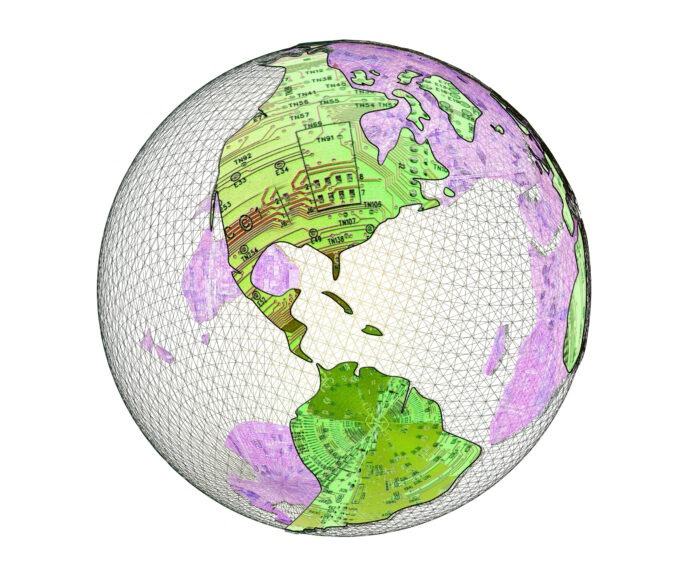# Mathematical Earth

0
87In mathematics 2 is the perfect number. From it comes the binary number system, from which all computers spring. In music there are eight notes in a set they are C, D, E, F, G, A, B and then C again. This sequence of eight is called an octave, oct being Greek for eight.

The Earth vibrates at a frequency of 7.83 cycles per second (Hz). Many believe that the natural frequency of the Earth is 8 Hz, but it has been slowed down by all negative actions of people. In music, middle C is 256 Hz. That is the sixth octave of eight cycles per second, the nearest whole number to the Earth frequency.

Tuning forks are used to bring the energy system into tune. There are several systems that are used. We have found two to be extremely effective. One is based on Kabalah, which we will not discuss here. The other uses the harmonic set.

The harmonic set matches the whole notes to the chakras. The root chakra is 256 Hz. And is middle C. The chakras are:

1 – C – 256 – Root

2 – D – 288 – Sacral

3 – E – 320 – Solar-plexus

4 – F – 341 – Heart

5 – G – 384 – Throat

6 – A – 427 – Third Eye

7 – B – 480 – Crown

Three feet above the crown is the transpersonal point. It is again a C, 512 Hz. that is an octave above middle C. Below the feet is the Earth Star, and it is one octave below middle C, 128 Hz.

In mathematics we can look at the powers of a number. That is the number that results from multiplying a number by itself. So the first power of 2 is 2, the second power of 2 is 4, the third 8. Eight is, again, the nearest whole number to the current frequency of the Earth, 7.83 Hz.

One little mathematical anomaly, what is the zero power of two? Two raised to the power zero is one. If you seek “nothingness” the result is “oneness”. In many of the mystical religious traditions they talk about getting to that state of nothingness where you become one with the universe.

In Kabalah we talk of this state as the point where there was no differentiation. Everything was one. There was no plus and minus, no good and evil, no sacred and profane. It is the first line in the bible “in the beginning there was God.”

The Dalai Lama talks about the same thing when he discusses meditation. He says that in the western traditions we have misinterpreted the goal of meditation. We say that the one who meditates attempts to calm the mind to get to a state of nothingness. On the contrary, you want to get to a state where your mind becomes one with the universe. That is, there is no differentiation between you and the universe.

We are attempting to find balance, calm, peace and oneness. We are all trying to find the source of all and become part of the one. Again, two to the zero power is one. If we reach the point where there are zero differences between us and the universe we find that the power of zero is oneness.

If we assume that mathematics is pure and two is the perfect number, is it not hard to extrapolate that the Earth should be vibrating to eight? Many psychics have been saying that to repair the world we need to raise the vibrational level, the frequency of the Earth. Let us take eight as the ideal vibration for the Earth.

Each power of two, that is each time we double the value, we go up one octave. Human hearing is from 20 to 20,000 cycles per second. The first octave in the human hearing range would be 32. Next are 64 and 128 and then comes middle C, 256, which is the frequency of the root chakra. We resonate to the Earth frequency of eight.